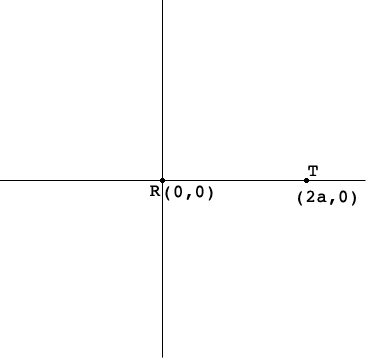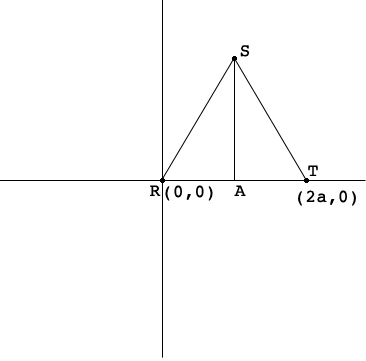SEARCH HOMEMath Central Quandaries & QueriesQuestion from Ethan: In equilateral triangle RST, R has coordinates (0, 0) and T has coordinates of (2a, 0). Find the coordinates of S in terms of a.Hi Ethan,

You haven't said if $a$ is positive or negative so I am going to assume that $a$ is positive.Again I don't know if $S$ is above or below the X-axis so I am going to assume it is above the X-axis.I added one point $A$ so that $SA$ is perpendicular to the X-axis.

What is the distance from $R$ to $A?$ What is the distance from $S$ to $A?$ Maybe Pythagoras can help. What are the coordinates of $S?$

Suppose that $S$ is below the X-axis. What are the coordinates of $S?$ Hence you have two possible answers for the question you were asked.

Now suppose $a$ is negative. Does that give you two more possible answers?

PennyMath Central is supported by the University of Regina and The Pacific Institute for the Mathematical Sciences.